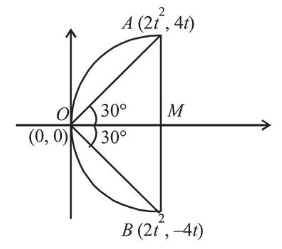# The area (in sq. units) of an equilateral triangle inscribedQuestion:

The area (in sq. units) of an equilateral triangle inscribed in the parabola $y^{2}=8 x$, with one of its vertices on the vertex of this parabola, is :

1. (1) $64 \sqrt{3}$

2. (2) $256 \sqrt{3}$

3. (3) $192 \sqrt{3}$

4. (4) $128 \sqrt{3}$

Correct Option: , 3

Solution:

Let $A=\left(2 t^{2}, 4 t\right)$ and $B=\left(2 t^{2},-4 t\right)$For equilateral triangle $\left(\angle A O M=30^{\circ}\right)$

$\tan 30^{\circ}=\frac{4 t}{2 t^{2}} \Rightarrow \frac{1}{\sqrt{3}}=\frac{4 t}{2 t^{2}} \Rightarrow t=2 \sqrt{3}$

Area $=\frac{1}{2} \cdot 8(2 \sqrt{3}) \cdot 2 \cdot 24=192 \sqrt{3}$.